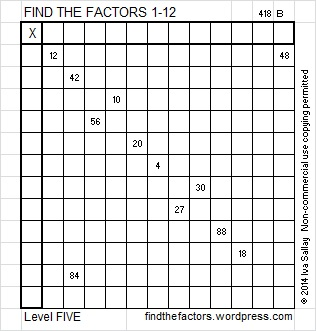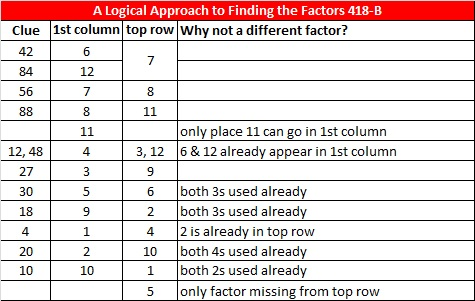# 113 and Level 5

• 113 is a prime number.
• Prime factorization: 113 is prime.
• The exponent of prime number 113 is 1. Adding 1 to that exponent we get (1 + 1) = 2. Therefore 113 has exactly 2 factors.
• Factors of 113: 1, 113
• Factor pairs: 113 = 1 x 113
• 113 has no square factors that allow its square root to be simplified. √113 ≈ 10.6301How do we know that 113 is a prime number? If 113 were not a prime number, then it would be divisible by at least one prime number less than or equal to √113 ≈ 10.6 Since 113 cannot be divided evenly by 2, 3, 5, or 7, we know that 113 is a prime number.

113 is never a clue in the FIND THE FACTORS puzzles.Excel file of puzzles and the previous week’s solutions: 12 Factors 2014-05-05This site uses Akismet to reduce spam. Learn how your comment data is processed.